﻿ 简单线性EV回归模型中最小二乘估计量的Berry-Esseen估计 A Note on LS Berry-Esseen Estimator in Simple Linear EV Regression Model

Vol.04 No.01(2015), Article ID:14858,7 pages
10.12677/AAM.2015.41004

A Note on LS Berry-Esseen Estimator in Simple Linear EV Regression Model

Jiao Meng, Mingming Yu

Nanjing University of Aeronautics and Astronautics, Nanjing Jiangsu

Received: Jan. 26th, 2015; accepted: Feb. 11th, 2015; published: Feb. 17th, 2015ABSTRACT

In this paper, we study the convergence rate of the central limit theorems for LS estimator in simple linear errors-in-variables (EV) regression model. Further, its application has been introduced detailedly by Miao, Yang and Shen in  .

Keywords:Central Limit Theorem, Convergence Rate, EV Regression Model, LS Estimator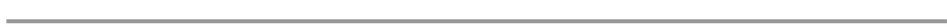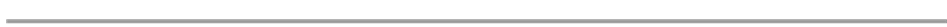1. 介绍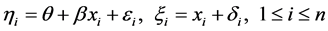(1)

(1)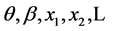是未知常数；

(2)是独立同分布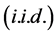随机变量，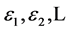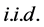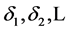，且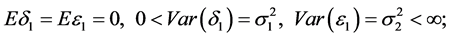(3)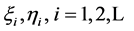是可观测值。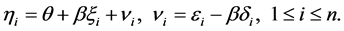(2)(3)(4)(5)(6)。因此，根据定理1.1有(2) 满足定理B的所有假设，我们知道。随即可以得到(1)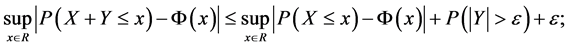(2)2. 定理的证明(7)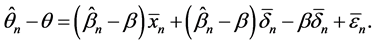(8)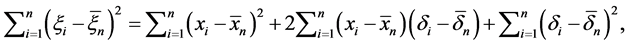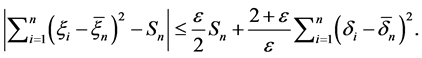(9)(10)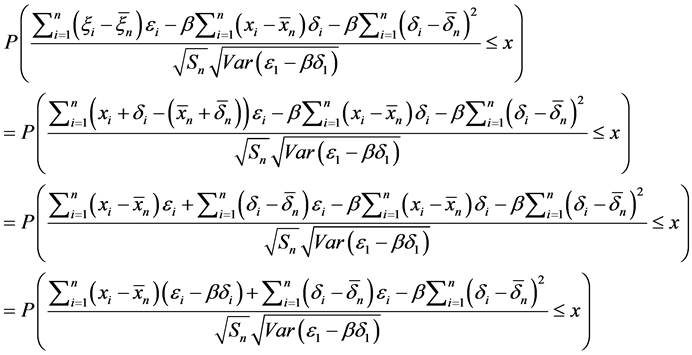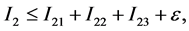(11)(12)，结合(9)，(10)，(11)，(12)，我们可以得到引理2.4的证明。(13)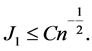(14)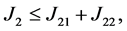(15)(16)，结合(13)，(14)，(15)，(16)和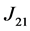的估计，我们可以证明引理2.5。(17)A Note on LS Berry-Esseen Estimator in Simple Linear EV Regression Model. 应用数学进展,01,29-36. doi: 10.12677/AAM.2015.41004

1. 1. Miao, Y., Yang, G.Y. and Shen, L.M. (2007) The central limit theorem for LS estimator in simple linear EV regression models. Communications in Statistics-Theory and Methods, 36, 2263-2272.

2. 2. Liu, J.X. and Chen, X.R. (2005) Consistency of LS estimator in simple linear EV regression model. Acta Mathematica Scientia, 25B, 50-58.

3. 3. Miao,Y. and Liu, W.A. (2009) Moderate deviations for LS estimator in simple linear EV regression model. Journal of Statistical Planning and Inference, 139, 3122-3131.

4. 4. Cui, H.J. (1997) Asymptotic normality of M-estimator in the EV model. Journal of System Science and Mathematics, 10, 225-236.

5. 5. Deaton, A. (1985) Panel data from a time series of cross-sections. Journal of Econometrics, 30, 109-126.

6. 6. Gleser, L.J. (1981) Estimation in a multivariate “error in variables” regression model: Large sample results. Annals of Statistics, 9, 24-44.

7. 7. Michel, R. and Pfanzagl, J. (1971) The accuracy of normal approximation for minimum contrast estimates. Zeitschrift fur Wahrscheinlichkeitstheorie und Verwandte Gebiete, 18, 73-84.

8. 8. Petrov, V.V. (1975) Sums of independent random variables. Springer, Berlin.